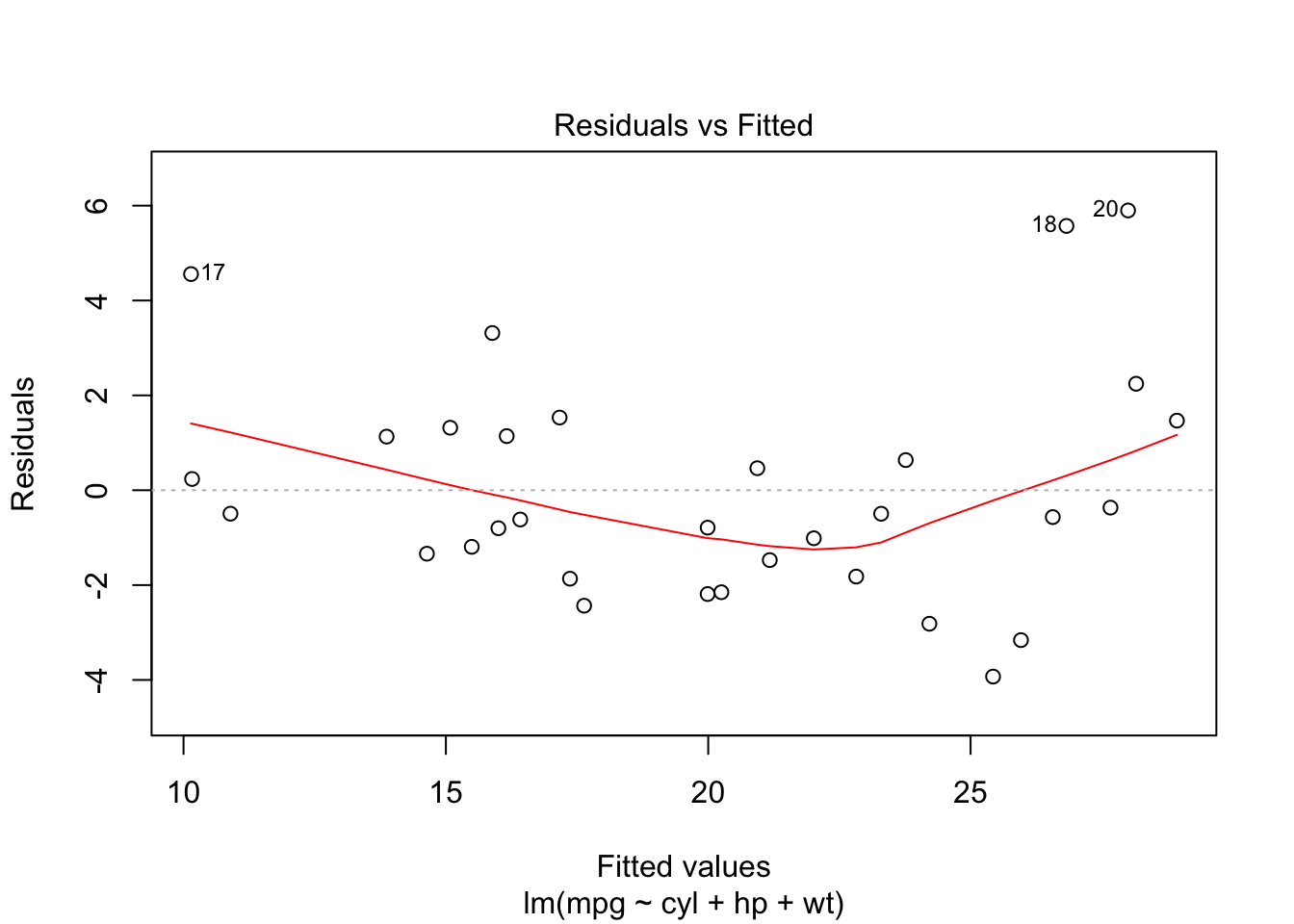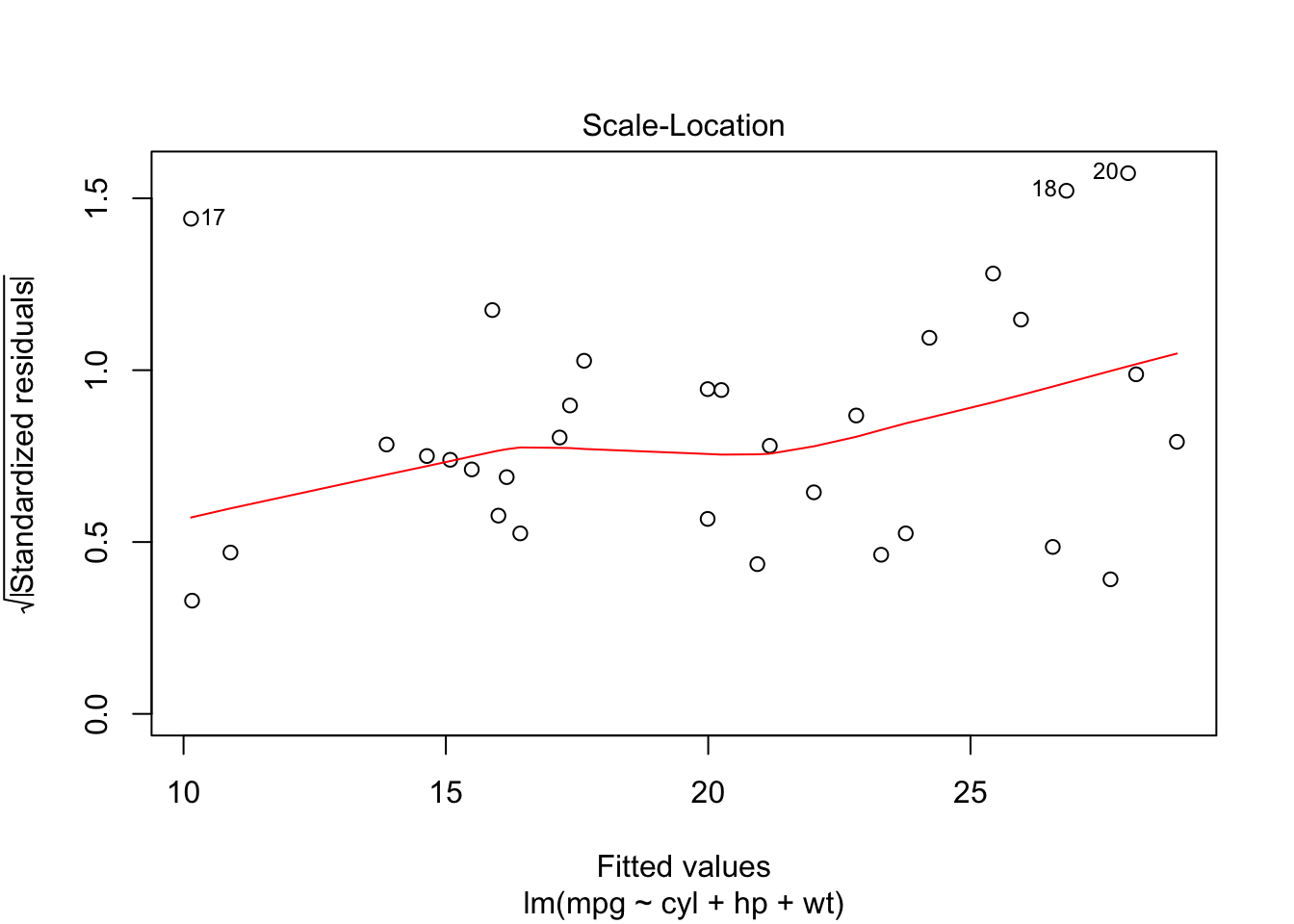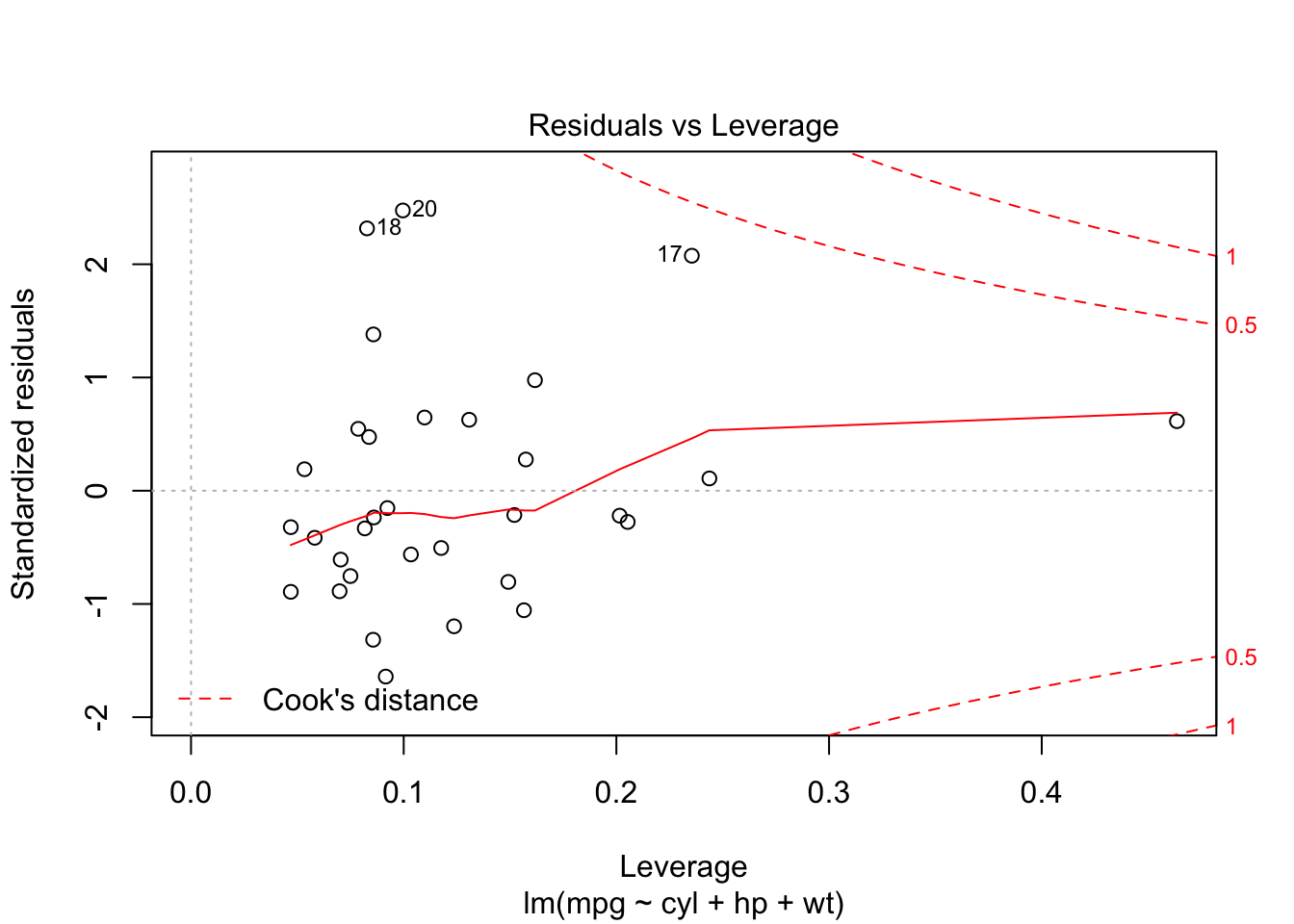## Multi-variate Regression

Multi-variate regression works in exactly the same way that simple regression works, with the exception that it is using more than one explanatory variable. This also means that it is not in two dimensional space, and is harder to picture mentally, although all the same ideas apply.

The basic equation for multi-variate regression is

$y=\beta_0+\beta_1 x_1+\beta_2 x_2+\dots+\beta_m x_m+e$

where $$\beta_0$$ is the intercept, $$\beta_1$$ is the slope for $$x_1$$, $$\beta_2$$ is the slope for $$x_2$$, etc. $$e$$ is still the error term. This regressions model is applied to $$n$$ observations, one from the response variable, and one each from the $$m$$ explanatory variables. Ideally pick values that you can justify based on practical or theoretical grounds. You could also choose variables that generate the largest value of Adjusted $$R^2$$, or you could choose those with the most significant p-values. Remember, when looking at the goodness-of-fit for multi-variate regression models, you must use Adjusted $$R^2$$!

Let’s try to conduct a multi-variate regression on the cars data. First, make sure that you’ve selected your dataAnalysis folder as your working directory. Now, read the cars data into R:

cars<-read.csv("cars.csv",as.is=TRUE)  ##Remember, the as.is=TRUE means that text data becomes character data 

Here’s a reminder of what each of the fields in this data set represent

Name Description
mpg Miles/(US) gallon
cyl Number of cylinders
disp Displacement (cu.in.)
hp Gross horsepower
drat Rear axle ratio
wt Weight (lb/1000)
qsec 1/4 mile time
vs V/S
am Transmission (0 = automatic, 1 = manual)
gear Number of forward gears
carb Number of carburetors

Now let’s set up our first multi-variate regression. Let’s choose variables that we think could impact the mileage of the car. To do this, let’s select cylinders, displacement, horsepower, weight, gear, and carburetor. Notice that we can add explanatory variables to the model with the $$+$$ symbol.

cars.lm<-lm(mpg~cyl+disp+hp+wt+gear+carb,data=cars)   ##Remember that lm stands for linear model

Now let’s look at a summary of this data:

summary(cars.lm)
##
## Call:
## lm(formula = mpg ~ cyl + disp + hp + wt + gear + carb, data = cars)
##
## Residuals:
##    Min     1Q Median     3Q    Max
## -3.363 -1.609 -0.451  1.521  5.875
##
## Coefficients:
##             Estimate Std. Error t value Pr(>|t|)
## (Intercept) 34.47662    8.24058    4.18  0.00031 ***
## cyl         -0.93081    0.81074   -1.15  0.26180
## disp         0.00881    0.01580    0.56  0.58233
## hp          -0.02203    0.02029   -1.09  0.28781
## wt          -3.23829    1.40689   -2.30  0.02996 *
## gear         1.09950    1.32828    0.83  0.41564
## carb        -0.37782    0.73130   -0.52  0.60994
## ---
## Signif. codes:  0 '***' 0.001 '**' 0.01 '*' 0.05 '.' 0.1 ' ' 1
##
## Residual standard error: 2.58 on 25 degrees of freedom
## Multiple R-squared:  0.853,  Adjusted R-squared:  0.817
## F-statistic: 24.1 on 6 and 25 DF,  p-value: 2.93e-09

Note that this output looks exactly like that of simple regression, except we have more entries in the “Coefficients” Table.

Let’s evaluate this model now. We start by looking at the Adjusted $$R^2$$ and see that, at 0.8173, it appears to have a strong fit. It is definitely stronger than the $$0.75$$ that we achieved using just weight in Lesson 3. We also notice that the p-value for the F-Statistic is very small indicating that we have a good model. Now we take a look at the p-values in the “Coefficients” table. This looks a little worrisome. We would like to see at least some of the variables under $$0.05$$. Note that only weight is below $$0.05$$, and some of them are very high, with number of carburetors coming in at $$0.6$$. Note that these p-values measure the significane of the variables in the presence of all of the other explanatory variables. This means we can’t just drop all of the variables with high p-values all at once, because these can change significantly if we just drop a single variable. We have to start dropping variables with high p-values one at a time. Let’s start by dropping carburetors and see what our model looks like then:

cars.lm2<-lm(mpg~cyl+disp+hp+wt+gear,data=cars)   ##Notice that we dropped carb
summary(cars.lm2)
##
## Call:
## lm(formula = mpg ~ cyl + disp + hp + wt + gear, data = cars)
##
## Residuals:
##    Min     1Q Median     3Q    Max
## -3.223 -1.686 -0.383  1.293  5.943
##
## Coefficients:
##             Estimate Std. Error t value Pr(>|t|)
## (Intercept)  37.3626     5.9725    6.26  1.3e-06 ***
## cyl          -1.1186     0.7144   -1.57   0.1295
## disp          0.0138     0.0123    1.12   0.2727
## hp           -0.0279     0.0166   -1.68   0.1052
## wt           -3.7143     1.0482   -3.54   0.0015 **
## gear          0.6788     1.0345    0.66   0.5175
## ---
## Signif. codes:  0 '***' 0.001 '**' 0.01 '*' 0.05 '.' 0.1 ' ' 1
##
## Residual standard error: 2.54 on 26 degrees of freedom
## Multiple R-squared:  0.851,  Adjusted R-squared:  0.822
## F-statistic: 29.7 on 5 and 26 DF,  p-value: 5.72e-10

Notice that our Adjusted $$R^2$$ went up, but that all of our p-values changed. This is the reason you have to drop variables one at a time. You can’t perceive all of the interactions between the variables in the model, and can’t predict exactly what will happen as you start dropping variables. Let’s try dropping one more variable. The highest p-value in the new model is gears, so we’ll drop gears in our next iteration.

cars.lm3<-lm(mpg~cyl+disp+hp+wt,data=cars)   ##Notice that we dropped gear
summary(cars.lm3)
##
## Call:
## lm(formula = mpg ~ cyl + disp + hp + wt, data = cars)
##
## Residuals:
##    Min     1Q Median     3Q    Max
## -4.056 -1.464 -0.428  1.285  5.827
##
## Coefficients:
##             Estimate Std. Error t value Pr(>|t|)
## (Intercept)  40.8285     2.7575   14.81  1.8e-14 ***
## cyl          -1.2933     0.6559   -1.97  0.05895 .
## disp          0.0116     0.0117    0.99  0.33139
## hp           -0.0205     0.0121   -1.69  0.10238
## wt           -3.8539     1.0155   -3.80  0.00076 ***
## ---
## Signif. codes:  0 '***' 0.001 '**' 0.01 '*' 0.05 '.' 0.1 ' ' 1
##
## Residual standard error: 2.51 on 27 degrees of freedom
## Multiple R-squared:  0.849,  Adjusted R-squared:  0.826
## F-statistic: 37.8 on 4 and 27 DF,  p-value: 1.06e-10

In this new model, we see marginal improvement in the adjusted $$R^2$$. Once again we see the p-values of our remaining coefficients change. You can see that this iterative methodology could be time consuming. This iterative methodology is what you would have to do if you were doing this in Excel (by the way, Excel makes it hard to drop variables, since to do so you have to completely delete or move the columns of your data). Luckily in R we have a function that will add and subtract variables for you and determine statistically what the best fitting model should be. The function we will use to do this is stepAIC. This function uses the Akaike information criterion (AIC) to measure the relative quality of a statistical model. AIC handles the trade-off between the goodness of fit of a model and the complexity of the model. The stepAIC function is found in the MASS package in R. The MASS package ships with R, so you don’t have to install it. It does not automatically load when you start R, however, and so you need to call library(MASS) before using the stepAIC function.

Below I show you how to use the stepAIC function. Notice that I’m using our original multi-variate model: cars.lm. This is because I want to give this stepAIC function a model with all possible variables, and let it drop variables until it arrives at the best model. The output of the function is the “winning” model. Note that I will assign the output to a variable name “winner”

library(MASS)
winner<-stepAIC(cars.lm)  ##Calculates my winning model

Now let’s look at the summary of this model:

summary(winner)
##
## Call:
## lm(formula = mpg ~ cyl + hp + wt, data = cars)
##
## Residuals:
##    Min     1Q Median     3Q    Max
## -3.929 -1.560 -0.531  1.185  5.899
##
## Coefficients:
##             Estimate Std. Error t value Pr(>|t|)
## (Intercept)  38.7518     1.7869   21.69   <2e-16 ***
## cyl          -0.9416     0.5509   -1.71   0.0985 .
## hp           -0.0180     0.0119   -1.52   0.1400
## wt           -3.1670     0.7406   -4.28   0.0002 ***
## ---
## Signif. codes:  0 '***' 0.001 '**' 0.01 '*' 0.05 '.' 0.1 ' ' 1
##
## Residual standard error: 2.51 on 28 degrees of freedom
## Multiple R-squared:  0.843,  Adjusted R-squared:  0.826
## F-statistic: 50.2 on 3 and 28 DF,  p-value: 2.18e-11

This gives us the statistical best model for this data. It has an adjusted $$R^2$$ of 0.8263, marginally better than cars.lm3. The final explanatory variables that were left in the model were cylinders, horespower, and weight.

Just like last lesson, we once again need to check our four regression assumptions. Remember that our assumptions were:

1. The relationship between the explanatory variables and the response variable is linear.
2. Errors in prediction of the value of Y are distributed in a way that approaches the normal curve.
3. Errors in prediction of the value of Y are all independent of one another. This means that all of the explanatory variables are independent of one another.
4. The distribution of the errors in prediction of the value of Y is constant regardless of the value of X (constant variance).

Let’s create our plots to check these assumptions:

plot(winner)From looking at our plots, we see that linearity and normality are close to being out of tolerance, but should be ok. We don’t seem to have any heteroscedasticity, or unequal variance. Looking at the Cook’s distance, we don’t see any outliers that will significantly affect our model. Finally, while our variables are somewhat related, we can assume we have enough independence that we can use our multiple-variate regression model.

## Homework

1. Import the NFL 2013 Season Team Data
2. Build a Multiple Variage Regression Model with Total Points as your response variable. Choose six explanatory variables (Don’t use all of the numeric variables! Since you only have 32 observations, you will lose degrees of freedom if you use too many explanatory variables.)
3. Print a summary of your model. Try iteratively dropping variables with high p-values. Does your model improve?
4. Use stepAIC to find the winning model using the first model that you developed in Step 2 above. What variables are in your “winning” model.
5. Check your final model to make sure it doesn’t violate any of our four regression assumptions.